Next: The Quantum Adversary: Up: Preliminaries Previous: Quantum Oracle Models   Contents

### A Lower Query Bound Proving Framework due to Ambainis

Ambainis  proved the following fundamental result:

Theorem 2.1.1 (Ambainis)   Let f be an N-bit Boolean function and let X and Y be two sets of inputs such that f (x)f (y) whenever x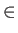X and yY. Let PX x Y be a relation between X and Y with the following properties:

1. Every element of X is related to at least m elements of Y by P.

2. Every element of Y is related to at least m' elements of X by P.

3. For all i, every element xX is related to at most l elements yY such that xiyi

4. For all i, every element yY is related to at most l' elements xX such that xiyi

Then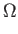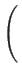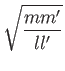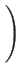oracle queries are required by any quantum algorithm to compute f in the bounded error setting.

This theorem will be the primary means of proving lower bounds in this thesis. Its appeal is twofold; first, it leads to reasonably simple proofs, and second, it unifies many existing lower bounds into a single framework.

SubsectionsNext: The Quantum Adversary: Up: Preliminaries Previous: Quantum Oracle Models   Contents
Matthew Hayward Lower Query Bounds in the Quantum Oracle Model GitHub Repository